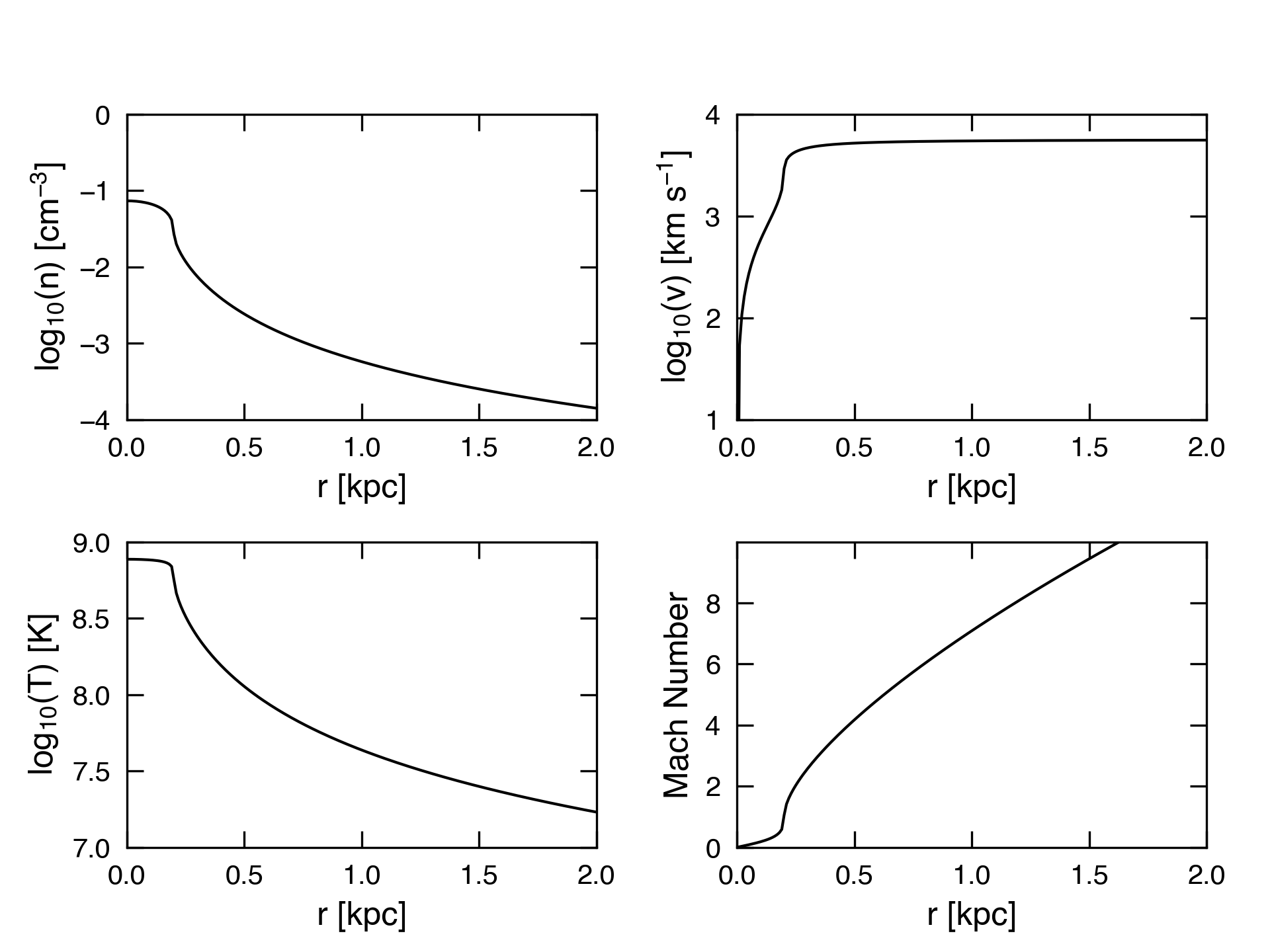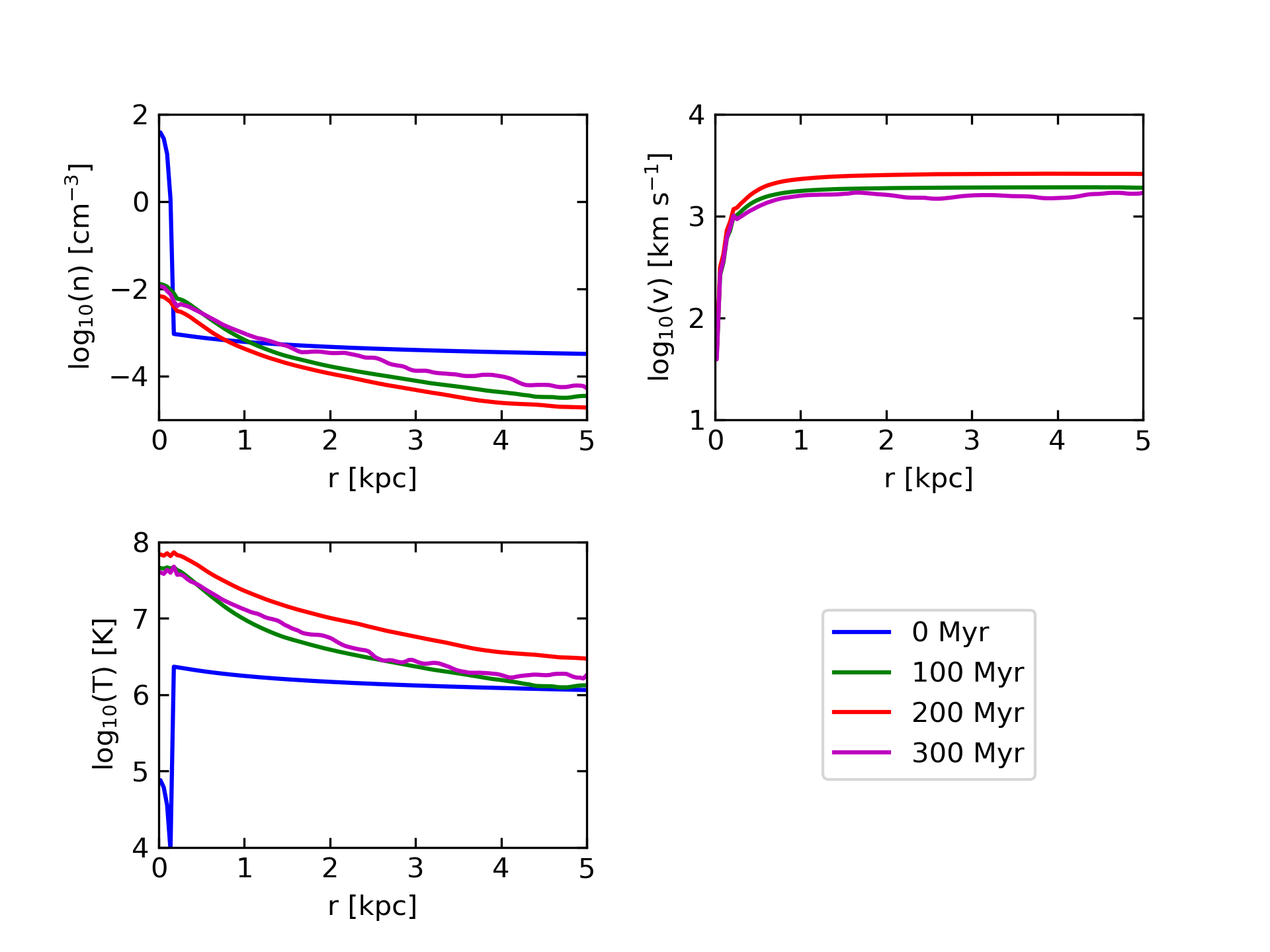# CC85 outflow

## June 12, 2017

At the end of the last post, I showed an M82 simulation with mass and energy input that failed to drive an outflow. The model parameters were set to match the CC85 model we used in our 2017 paper: $R_{*} = 300$pc, $\dot{M}_{\odot} = 2 M_{\odot}$/yr, and $\dot{E} = 10^{42}$erg/s. At all times during the simulation, a small bubble of heated disk gas can be seen near the starburst region, but the bubble never propagates into the halo.

I concluded that the gas density in the center was too high for the energy input to superheat the gas, and the outflow was suppressed. To rectify this, I have now run a simulation with significantly more extreme feedback parameters. Namely, I chose the parameters given in the initial Chevalier & Clegg (1985) paper, with $\beta = \alpha = 1$. These are $R_{*} = 200$pc, $\dot{M}_{\odot} = 1 M_{\odot}$/yr, and $\dot{E} = 10^{43}$erg/s. Recall that $\beta$ in this context means mass-loading of the hot phase beyond mass from supernova ejecta, and $\alpha$ refers to the thermalization fraction. These numbers are based on an assumption that M82 experiences $\sim3$ supernovae every 10 years, and the energy production of a single supernova is $10^{51}$erg. The analytic solution for an outflow with these parameters is shown in the plot below.Using the same M82 initial conditions as described in the last post, I was able to run a $256^3$ simulation with the above mass and energy input rates for 327 Myr, at which time the simulation crashed. Below are movies showing density and density-weighted temperature projections, as well as temperature slices through the y and z midplanes for the simulation. The simulation uses PPMC, the exact Riemann solver, the Van Leer integrator, and no-inflow boundary conditions. Mass and energy input begins at 10 Myr.

As the movies clearly show, the more extreme parameters do result in an outflow. Within the first 50 Myr, a bubble of high density disk gas is pushed out into the halo and driven out of the box. Once the high density gas has been cleared out of the center, the outflow stabilizes for 200 Myr or so, with a fairly narrow cone of high temperature gas ($T > 10^{6.5}$K) surrounded by a lower temperature interface ($T\sim10^{4.5}$K). After 250 Myr, the outflow becomes unstable, eventually leading to the crash (a negative temperature cell can be seen in the upper left of the temperature slice movie in the last frame). Interestingly, even during the stable period, the outflow never reaches the temperatures expected from the CC85 solution. Compare the plot below, which shows density, z-velocity, and temperature along the $(x, y) = (0, 0)$ axis, to the plot at the beginning of this post.It is worth noting that this simulation took MUCH longer than the previous versions. The higher temperatures near the center resulted in a fairly small timestep, which meant that even with a cfl coefficient of 0.4, running to 327 Myr with $128^3$ cells / GPU took around 8.5 hours. If we were to extrapolate that to a $2048^3$ simulation running on 4096 GPUs, the simulation would take 68 hours, or 8.4 million core-hours (likely a bit longer, since our weak scaling isn’t perfect). When we did the calculations for our INCITE proposal, we were assuming a timestep based on hot gas temperatures of $10^{6.5}$ K. That said, when doing the calculations for the proposal, we also assumed a cfl coefficient of 0.1. As it turns out, we estimated a time of 9 million core-hours to run a quandrant simulation (1024x1024x4096) for 400 Myr.